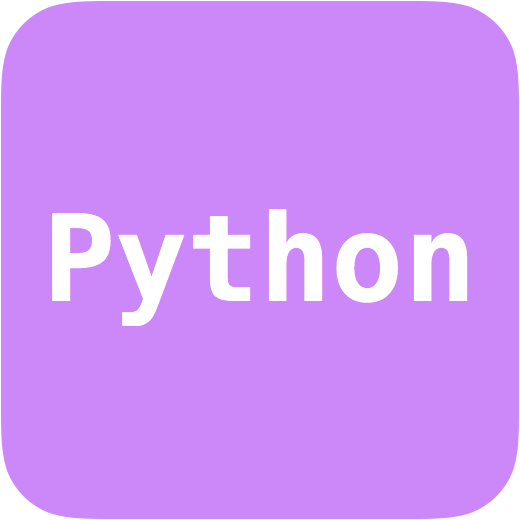# SF_DeconvolveAuthors: S. Farrens Language: Python Download: GitHub Description: A Python code designed for PSF deconvolution using a low-rank approximation and sparsity. The code can handle a fixed PSF for the entire field or a stack of PSFs for each galaxy position. Notes: This code was used to produce the results presented in the paper Space variant deconvolution of galaxy survey images. Sample Euclid-like PSF data can be downloaded from here [63Mb] Sample galaxy images can be downloaded from here [5.5Mb]

## Introduction

The following sections provide some details for how to run sf_deconvolve.

The directory lib contains all of the primary functions and classes used for optimisation and analysis. functions contains some additional generic functions and tools.

## Dependencies

In order to run the code in this repository the following packages must be installed:

• Python 2.7 [Tested with v 2.7.11]
• Numpy [Tested with v 1.11.3]
• Scipy [Tested with v 0.18.1]
• Astropy [Tested with v 1.1.2]
• Matplotlib [Tested with v 1.5.3]
• Termcolor [Tested with v 1.1.0]

The current implementation of wavelet transformations additionally requires the mr_transform.cc C++ script from the Sparse2D library in the iSap package [Tested with v 3.1]. These C++ scripts will be need to be compiled in order to run (see iSap Documentation for details).

The low-rank approximation method can be run purely in Python.

## Execution

The primary code is sf_deconvolve.py which is designed to take an observed (i.e. with PSF effects and noise) stack of galaxy images and a known PSF, and attempt to reconstruct the original images. The input format are Numpy binary files (.npy) or FITS image files (.fits).

The code can be run as follows:

`\$ sf_deconvolve.py -i INPUT_IMAGES.npy -p PSF.npy -o OUTPUT_NAME`

Where INPUT_IMAGES.npy denotes the Numpy binary file containing the stack of observed galaxy images, PSF.npy denotes the PSF corresponding to each galaxy image and OUTPUT_NAME specifies the output path and file name.

Alternatively the code arguments can be stored in a configuration file (with any name) and the code can be run by providing the file name preceded by a @.

`\$ sf_deconvolve.py @config.ini`

### Example

The following example can be run on the sample data provided in the example directory.

This example takes a sample of 100 galaxy images (with PSF effects and added noise) and the corresponding PSFs, and recovers the original images using low-rank approximation via Condat-Vu optimisation.

```\$ sf_deconvolve.py -i example_image_stack.npy -p example_psf.npy -o example_output --mode lowr

```

The example can also be run using the configuration file provided.

The result will be two Numpy binary files called example_output_primal.npy and example_output_dual.npy corresponding to the primal and dual variables in the splitting algorithm. The reconstructed images will be in the example_output_primal.npy file.

### Code Options

Required Arguments

-i INPUT, --input INPUT: Input data file name. File should be a Numpy binary containing a stack of noisy galaxy images with PSF effects (i.e. a 3D array).

-p PSF, --psf PSF: PSF file name. File should be a Numpy binary containing either: (a) a single PSF (i.e. a 2D array for fixed format) or (b) a stack of PSFs corresponding to each of the galaxy images (i.e. a 3D array for obj_var format).

Optional Arguments

-h, --help: Show the help message and exit.

-v, --version: Show the program's version number and exit.

-q, --quiet: Suppress verbose for each iteration.

-o, --output: Output file name. If not specified output files will placed in input file path.

--output_format Output file format [npy or fits].

Initialisation

-k, --current_res: Current deconvolution results file name (i.e. the file containing the primal results from a previous run).

--noise_est: Initial estimate of the noise standard deviation in the observed galaxy images. If not specified this quantity is automatically calculated using the median absolute deviation of the input image(s).

Optimisation

-m, --mode {all,sparse,lowr,grad}: Option to specify the optimisation mode [all, sparse, lowr or grad]. all performs optimisation using both low-rank approximation and sparsity, sparse using only sparsity, lowr uses only low-rank and grad uses only gradient descent. (default: lowr)

--opt_type {condat,fwbw,gfwbw}: Option to specify the optimisation method to be implemented [condat, fwbw or gfwbw]. condat implements the Condat-Vu proximal splitting method, fwbw implements Forward-Backward splitting with FISTA speed-up and gfwbw implements the generalised Forward-Backward splitting method. (default: condat)

--n_iter: Number of iterations. (default: 150)

--cost_window: Window to measure cost function (i.e. interval of iterations for which cost should be calculated). (default: 1)

--convergence: Convergence tolerance. (default: 0.0001)

--no_pos: Option to turn off positivity constraint.

Low-Rank Aproximation

--lowr_thresh_factor: Low rank threshold factor. (default: 1)

--lowr_type: Type of low-rank regularisation [standard or ngole]. (default: standard)

--lowr_thresh_type: Low rank threshold type [soft or hard]. (default: hard)

Sparsity

--wavelet_type: Type of Wavelet to be used (see iSap Documentation). (default: 1)

--wave_thresh_factor: Wavelet threshold factor. (default: [3.0, 3.0, 4.0])

--n_reweights: Number of reweightings. (default: 1)

Condat Algorithm

--relax: Relaxation parameter (rho_n in Condat-Vu method). (default: 0.8)

--condat_sigma: Condat proximal dual parameter. (default: 0.5)

--condat_tau: Condat proximal primal parameter. (default: 0.5)

Testing

-c, --clean_data: Clean data file name.

-r, --random_seed: Random seed. Use this option if the input data is a randomly selected subset (with known seed) of the full sample of clean data.

--kernel: Standard deviation of pixels for Gaussian kernel. This option will multiply the deconvolution results by a Gaussian kernel.

--metric: Metric to average errors [median or mean]. (default: median)

## Troubleshooting

If you get the following error:

```ERROR: svd() got an unexpected keyword argument 'lapack_driver'

```

Update your Numpy and Scipy installations

```\$ pip install --upgrade numpy